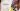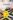# How To Play Easy Math Games Using Dice

Math games let kids practice and consolidate math skills in a non-threatening and highly motivational way. Today I’m sharing easy math games using dice.Math games let kids practice and consolidate math skills in a non-threatening and highly motivational way. You can find tons of online math games, but most of my favorite math games are decidedly low tech. You don’t need much more than a deck of cards or some dice to play many fun math games. Today I’m sharing easy math games using dice.

## 3 easy math games using dice

Kids may not know that dice helps develop skills in number sense, problem solving, classifying, and probability. They do know that dice are a lot of fun. There’s just something about dice that kids seem to love. So bring out the dice, and bring out the fun and learning.

You’ll need dice (2 or 3 per student or group), number grid 1–10, paper and pencil for recording results.

## Just 100

Use this game with students who are working on addition and subtraction. The goal is to get to exactly 100. Students roll a die and add or subtract the number until they get to 100. They can do the math mentally or you can have them show each equation. You can try with two dice as well. Students can decide to add both, subtract both or add one and subtract the other.

## Knock Out

Give each student a number grid 1–10 or have them create one on paper. Each student also needs two dice and a pencil. The goal is to cross out each number on their grid.

To play, have each student roll both dice. They can use any operation (addition, subtraction, multiplication, or division) to get one of the numbers. For example, if you roll 6 and 3, you could knock out 9, 3, or 2.

6 + 3 = 9

6 – 3 = 3

6 / 3 = 2

(6 * 3 = 18, which is too high to be on the grid)

You can easily adapt this game for younger students by limiting it to addition and subtraction.

To expand on this game, ask students to predict which numbers will be most challenging to get. Then ask how many ways they can find to get a particular number.

## Top 3

This is another game you can modify easily for different levels. Each student or team will need 3 dice and paper and pencil for tracking.

The goal is to reach a preset number (100, 1000, or some other number you set). Students roll 3 dice and set the highest number aside. For example, if they roll 5, 3, 2, they would set the 5 aside.

They roll the other two, and again set the highest number aside. They roll the third die.

Students add up the three numbers. Then they roll again and continue in the same way. They’ll add their second sum to the first and keep going until they get to a certain number (100, or 1000).

You can also change up the rules to include other operations. For example, add the first two dice and then multiply by the third number, or multiply the first two and then subtract the third.

I hope your kiddos have fun with these easy math games. What easy math games with dice do you play in your class?

## FREE easy math games

Looking for more easy math games. Get 3 free printables.

Fill in the form below for 2D shape dominoes, transformation puzzles, and estimating game.## Why I Love Task Cards For Teaching Multiplication

Teaching multiplication goes beyond having children memorize multiplication tables. To build math fluency, try these best ways to teach multiplication.

## 6 Great Ideas For Teaching Place Value

Teaching place value is important for students. Place value helps us to read whole numbers into the millions and beyond. Try these place value activities.